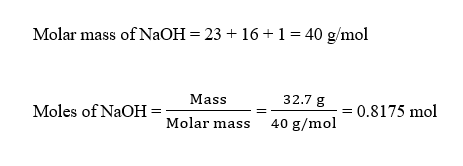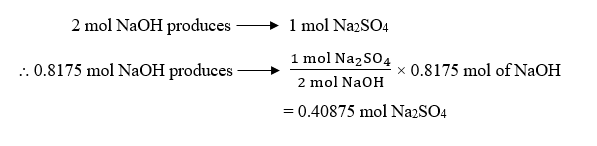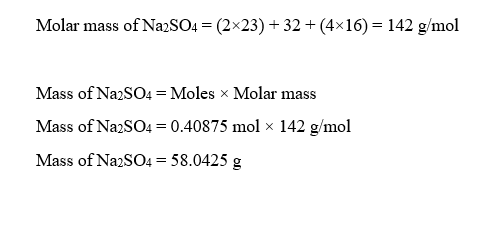# For the reaction2NaOH+H2SO4⟶Na2SO4+2H2O2NaOH+H2SO4⟶Na2SO4+2H2Ohow many grams of sodium sulfate, Na2SO4, are produced from 32.732.7 g of sodium hydroxide, NaOH?mass of sodium sulfate:

Question
2 views

For the reaction

2NaOH+H2SO4⟶Na2SO4+2H2O2NaOH+H2SO4⟶Na2SO4+2H2Ohow many grams of sodium sulfate, Na2SO4, are produced from 32.732.7 g of sodium hydroxide, NaOH?

mass of sodium sulfate:
check_circle

Step 1

Given,

Mass of NaOH = 32.7 g

Moles of NaOH can be calculated as:Step 2

The balanced chemical reaction is given as:

2 NaOH + H2SO4 → Na2SO4 + 2 H2O

From the given reaction, it is evident that :Mass of Na2SO4 produced can be calculated as:...

### Want to see the full answer?

See Solution

#### Want to see this answer and more?

Solutions are written by subject experts who are available 24/7. Questions are typically answered within 1 hour.*

See Solution
*Response times may vary by subject and question.
Tagged in